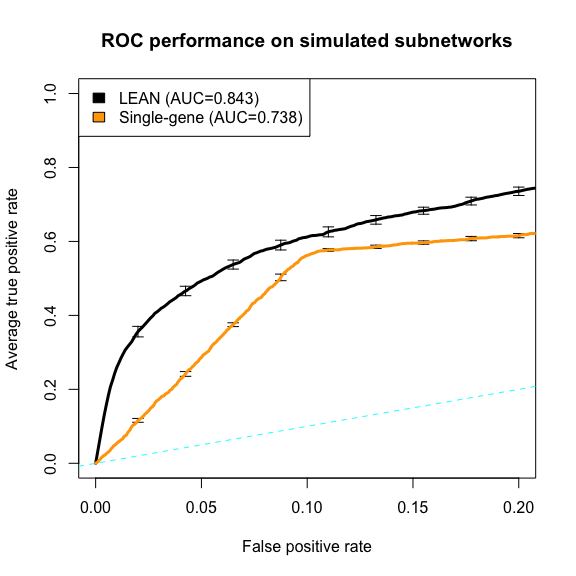# Run LEAN on simulated subnetwork data

library(LEANR)
library(ROCR)
set.seed(123456)
data(g2)

Create 10 instances of simulated subnetworks and gene p-values with default parameters: Number of modules = 10; Module size between 10 and 50; p_scale = 0.1; p_enrich = 0.5; with preferential attachment

n_instances=10

instances<-lapply (1:n_instances,function(i){
subnet.simulation(g2, spec=sprintf('_STRING.fs900_rep%i',i), create.files=F)
})

Run LEAN on the subnetwork simulation instances. 1000 background permutations are enough for the purpose of the subnetwork simulation study. When we run these calculations for 10 separate subnetwork simulation instances, it still takes about 10 * 1.5 = 15 minutes (using 3 cores).

LEAN_results_sim<-lapply(1:n_instances,function(i){
pvals<-instances[[i]]$pvals[,'P.Value'] names(pvals)<-rownames(instances[[i]]$pvals)
run.lean.fromdata(pvals, g2, n_reps = 1000, ncores = 3)
})

Determine performance of LEAN and single-gene scoring on the subnetwork simulation data

# extract gene class labels for each of the simulation instances
gene.order<-rownames(instances[]$pvals) class_matrix<-t(foreach(i=1:length(instances),.combine=rbind) %do% { tmp<-!grepl('BG',instances[[i]]$pvals$NodeType); names(tmp)<-rownames(instances[[i]]$pvals);
tmp[gene.order]
})

# extract LEAN scores for each of the simulation instances
LEAN_matrix<-t(foreach(i=1:length(instances),.combine=rbind) %do% {
tmp<-LEAN_results_sim[[i]]$restab[,'pstar'] names(tmp)<-rownames(LEAN_results_sim[[i]]$restab);
tmp[gene.order]
})

# Extract single gene scores
SG_matrix<-t(foreach(i=1:length(instances),.combine=rbind) %do% {
tmp<-instances[[i]]$pvals[,'P.Value'] names(tmp)<-rownames(instances[[i]]$pvals);
tmp[gene.order]
})

# ROC evaluation
predictions<-list(LEAN=prediction(-LEAN_matrix,class_matrix),
SG=prediction(-SG_matrix,class_matrix))

performances<-lapply(predictions,function(pred){performance(pred,"tpr","fpr")})
names(performances)<-names(predictions)

aucs_ind<-lapply(predictions,function(pred){performance(pred,"auc")@y.values})
aucs<-lapply(aucs_ind,function(x)mean(unlist(x)))
names(aucs)<-names(predictions)

Plot ROC curves

# plot resulting ROC curves
plot(performances$LEAN,xlim=c(0,.2),col='black',avg='vertical',lwd=3, spread.estimate='stderror',show.spread.at=seq(.02,.2,l=9),main='ROC performance on simulated subnetworks') plot(performances$SG,col='orange',avg='vertical',lwd=3,add=T,
legend('topleft',c(sprintf('LEAN (AUC=%0.3g)',aucs$LEAN),sprintf('Single-gene (AUC=%0.3g)',aucs$SG)),fill=c('black','orange'))